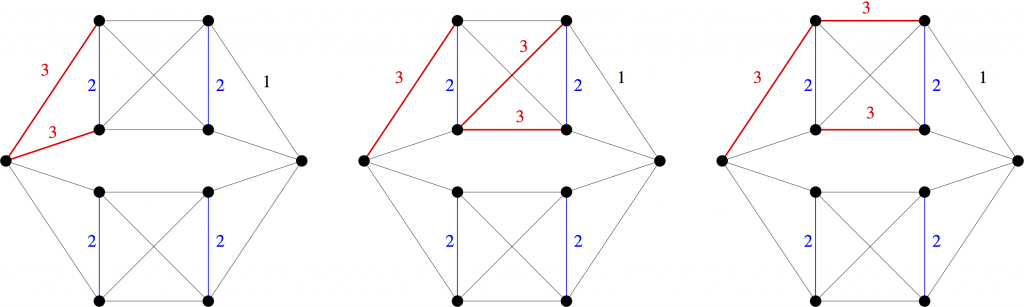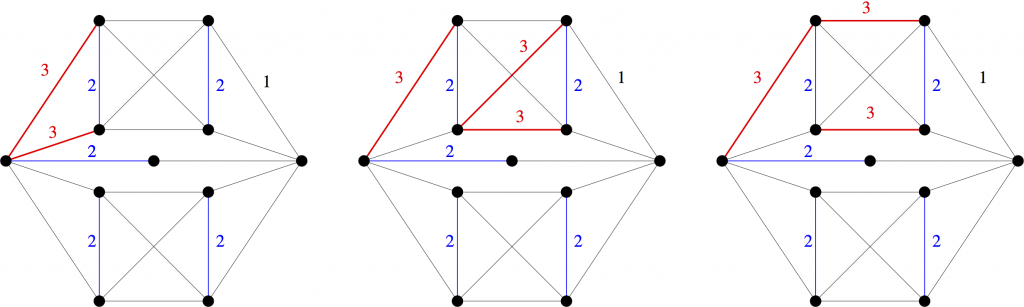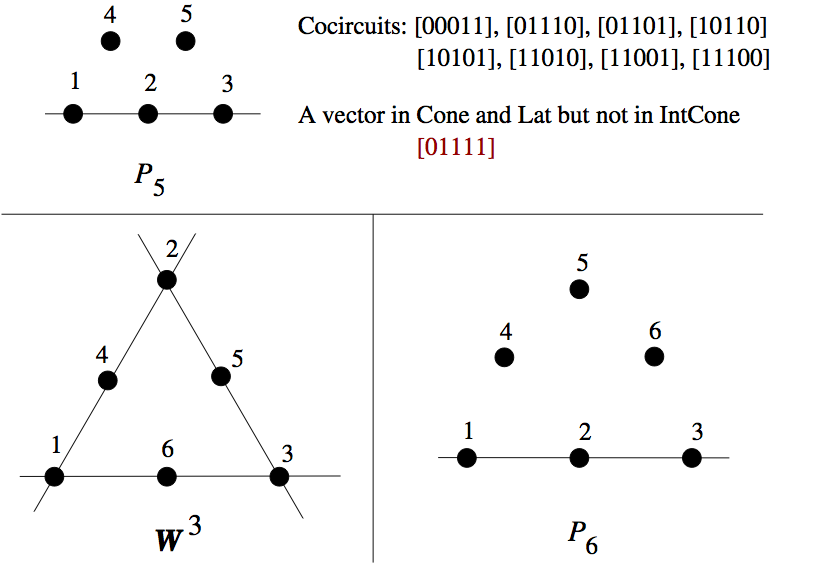# Hilbert Bases of Cuts and Cocircuits

Let $X$ be a set of vectors in $\mathbb{R}^d$.  Three important objects related to $X$ are the cone, lattice, and integer cone generated by $X$.  These are (respectively), the set of all real non-negative combinations, the set of all integer combinations, and the set of all non-negative integer combinations of vectors in $X$.  Clearly, the integer cone is contained in the intersection of the cone and the lattice.  If the integer cone is equal to the intersection of the cone and the lattice, we say that $X$ is a Hilbert basis.

Hilbert bases were introduced by Giles and Pulleyblank  as a tool to study total dual integrality. They are often nicer to work with than arbitrary sets of vectors.  For example, from the computational perspective, membership testing can be much easier for the cone and lattice than for the integer cone.

Given a graph $G$ (or matroid), we may regard subsets of $E(G)$ as characteristic $(0,1)$-vectors. In this way, we can ask whether a family $\mathcal{S}$ of subgraphs of $G$ is a Hilbert basis. In the case that $\mathcal{S}$ is the set of circuits of $G$, there is the following nice theorem of Alspach, Goddyn and Zhang .

Theorem 1 [Alspach, Goddyn, Zhang].  The set of circuits of a graph $G$ is a Hilbert basis if and only if $G$ does not contain the Peterson graph as a minor.

From the matroidal perspective, the difference between circuits and cuts is superficial, so it is very natural to ask the following question.

Question 2.  For which graphs $G$ is the set of cuts of $G$ a Hilbert basis?

Let us define a graph to be cut- (respectively circuit-) Hilbert if its set of cuts (respectively circuits) is a Hilbert basis.  Let $K_{5}^{\perp}$ be the unique 3-connected uncontraction of $K_5$.

Laurent  had previously shown that $K_{5}^{\perp}$ is cut-Hilbert.

In a recent paper of Deshpande, Goddyn and myself , we prove that all graphs without a $K_{5}^{\perp}$-minor are cut-Hilbert.

Theorem 3 [Deshpande, Goddyn, H].  Every graph without a $K_{5}^{\perp}$-minor is cut-Hilbert.

However, somewhat curiously, we also prove that the class of cut-Hilbert graphs is not as well-behaved as the class of circuit-Hilbert graphs.

Theorem 4 [Deshpande, Goddyn, H].  The class of cut-Hilbert graphs is not closed under edge-deletion, edge-subdivision, nor 2-sums.

Note that Theorem 4 is in stark contrast to Theorem 1, where there is an exact excluded-minor characterization for the class of circuit-Hilbert graphs.

I will now give the examples that establish Theorem 4.

It will be important to clarify what we mean by a 2-sum.  A 2-sum of two graphs is obtained by gluing them together along a common edge, and then deleting that edge. A 2-clique-sum of two graphs is obtained by gluing them together along a common edge, but keeping that edge. In contrast to 2-sums, Laurent  also proved that the family of cut-Hilbert graphs is closed under 2-clique-sums.Three different weightings of a non-cut-Hilbert graph $H_1$. Black edges all have weight 1.

Consider the three edge-weightings of the above graph $H_1$.  For a simple graph $G$, it is easy to show that a vector $x \in \mathbb{Z}^{E(G)}$ is in the lattice of cuts of $G$ if and only if $x(C)$ is even for every circuit $C$ of $G$.  One easily checks that this condition holds for each of the above three edge-weightings.  Furthermore, one can also write (but for the benefit of the reader we will not) each of these vectors as real non-negative combinations of cuts of $H_1$. On the other hand, none of these vectors is in the integer cone of cuts of $H_1$ (this is just a (large) finite case check).  This last claim was verified by our silent robot partner Normaliz , but can also be checked by humans (as we do in the paper). Therefore, $H_1$ is not cut-Hilbert.Three different weightings of a non-cut-Hilbert graph $H_2$. Black edges all have weight 1.

Similarly, each of the three edge-weightings of the above graph $H_2$ show that $H_2$ is not cut-Hilbert. Now let $H_3$ be the 2-clique-sum of two copies of $K_{5}^{\perp}$ along the distinguished edge $e$. Since $H_3$ is a 2-clique-sum of cut-Hilbert graphs, $H_3$ is also cut-Hilbert. However, note that $H_1$ is obtained from $H_3$ by deleting an edge and $H_2$ is obtained from $H_3$ by subdividing an edge. This establishes Theorem 4, as promised.

I will finish by discussing the matroid analogue of Question 2.

Question 5.  For which matroids $M$ is the set of cocircuits of $M$ a Hilbert basis?

Luis Goddyn and Marko Mitrovic (an NSERC undergraduate research student of Luis in 2013) made some interesting progress which I will briefly report here. Using Normaliz, the matroid Sage package, and the catalogue of all matroids up to 9 elements, they were able to determine all the non-cocircuit-Hilbert matroids up to 8 elements.  It turns out that there are lots of them.  In particular, of the 2198 matroids with up to 8 elements, 1305 are non-cocircuit-Hilbert.  Below is an image of the three smallest non-cocircuit-Hilbert matroids.If one restricts to binary matroids, then the situation is more manageable. There are only two binary non-cocircuit-Hilbert matroids up to 8 elements, and interestingly, both of them are coextensions of the Fano matroid.  Therefore, the following problem may be doable.

Problem 6.  Characterize the binary matroids whose set of cocircuits is a Hilbert basis.

Note that Problem 6 is open even for graphs.  By Theorem 4, the set of cut-Hilbert graphs is not closed under taking minors, so it does not have an excluded-minor characterization. However, perhaps there is an alternate nice characterization.

References

 F. R. Giles and W. R. Pulleyblank.  Total dual integrality and integer polyhedra.  Linear Algebra Appl., 25:191-196, 1979.

 Brian Alspach, Luis Goddyn, and Cun Quan Zhang.  Graphs with the circuit cover property. Trans. Amer. Math. Soc., 344(1):131-154, 1994.

 Monique Laurent.  Hilbert bases of cuts.  Discrete Math., 150(1-3):257-279, 1996.

 Tanmay Deshpande, Luis Goddyn, and Tony Huynh.  On Hilbert bases of cuts. arXiv.

 Winfred Bruns and Bogdan Ichim.  Normaliz: algorithms for affine monoids and rational cones.  J. Algebra, 324(5):1098-1113, 2010.

## SageMath again in Google Summer of Code

### Aside

SageMath has, once again, be selected as one of the mentoring organizations for the Google Summer of Code. This means any student, undergrad or PhD anywhere in the world, can spend the summer improving and extending the functionality of SageMath, while being paid by Google.

You should hurry, because the deadline is March 27.

# Seymour’s 1-flowing conjecture III

A long time ago, I gave some background to the definition of a 1-flowing matroid. Assume $M$ is a matroid on the ground set $E$, and $e$ is an element in $E$. Take $c_{x}$ to be a non-negative integral capacity assigned to each element, $x$, in $E-e$. A flow is an assignment of a non-negative real, $f_{C}$, to each circuit, $C$, that contains $e$, with the constraint that if $x$ is in $E-e$, then the sum of $f_{C}$ over all circuits that contain $e$ and $x$ does not exceed $c_{x}$. We say that $M$ is $e$-flowing if, for every assignment of capacities, there is a flow whose value (sum over all circuits containing $e$) is equal to
$\min\left\{\sum_{x\in C^{*}-e} c_{x}\mid C^{*}\ \text{is a cocircuit containing}\ e\right\}.$
If $M$ is $e$-flowing for every $e$ in $E$, then $M$ is $1$-flowing.

We are motivated to study $1$-flowing matroids because problems that should really be intractable, magically become easy to solve when we consider such matroids. Consider the case of a graph with distinct vertices $s$ and $t$. We might ask for the largest number of edge-disjoint paths from $s$ to $t$. Now this resembles a difficult problem: we are asking to find a largest-possible pairwise-disjoint subfamily from a family of sets (the edge-sets of $s-t$ paths). Finding the maximum number of pairwise-disjoint sets is famously an NP-hard problem. However, as is well known, the problem of finding edge-disjoint $s-t$ paths can be solved using network-flow techniques by assigning a capacity of one to every edge. This really reflects the fact (discussed earlier) that graphic matroids are $1$-flowing. The fact that the maximum value of a flow through a network is equal to the minimum capacity of a cut gives enough structure to the problem to render it feasible.

In my most recent post, we can see another intimation that difficult problems may become tractable when dealing with $1$-flowing matroids. Let $M$ be a matroid on the ground set $E$, where $e$ is an element in $E$. We assign a real-valued variable $a_{x}$ to each element $x\in E-e$, and we insist that $a_{x}$ takes only non-negative values. In addition, for each circuit, $C$, that contains $e$, we require that the sum of variables, taken over all elements in $C-e$, must be at least one. Consider the set, $P$, of points in the Euclidean space $\mathbb{R}^{E-e}$ corresponding to assignments of values to variables such that these constraints are satisfied. Since $P$ is an intersection of half-spaces, it is a polyhedron. Now $M$ is $e$-flowing if and only if the $0$-dimensional extreme points (that is, the vertices) of $P$ have entirely integral coordinates. This means that integer programming problems associated with $P$ are in fact amenable to linear programming techniques. In other words, if there is a linear objective function which assigns a cost to each point in $P$, and we wish to find lowest-cost point in $P$ with integer coordinates, then we can relax the integrality constraint, since running the unconstrained linear program will return an integral point in any case. As integer programming is $NP$-hard, and linear progamming is solvable in polynomial time, this gives us some intuition as to why ostensibly difficult problems are easy for $1$-flowing matroids.

Proposition. The class of $1$-flowing matroids is closed under minors.

Proof. Let $M$ be a $1$-flowing matroid on the ground set $E$, and let $e$ be an element in $E$. Let $f$ be in $E-e$. We will show that $M\backslash f$ is $e$-flowing. Assume that each element $x\in E(M\backslash f)-e$ has been assigned a capacity $c_{x}$. We assign exactly the same capacities to these elements in $M$, and in addition we assign $f$ a capacity of $0$. Now every cocircuit of $M\backslash f$ is of the form $C^{*}-f$ where $C^{*}$ is a cocircuit of $M$, or is equal to a cocircuit of $M$. It follows that
$\min\left\{\sum_{x\in C^{*}-e} c_{x}\mid e\in C^{*}\in\mathcal{C}^{*}(M)\right\}\leq\min\left\{\sum_{x\in C^{*}-e} c_{x}\mid e\in C^{*}\in\mathcal{C}^{*}(M\backslash f)\right\}.$
If this is a strict inequality, then there is some cocircuit $C^{*}$ in $M$ that contains $e$, where the sum of capacities in $C^{*}-e$ is less than the minimum such sum in $M\backslash f$. Obviously $C^{*}$ is not a cocircuit of $M\backslash f$, so $f$ is in $\operatorname{cl}^{*}(C^{*})$. Thus some cocircuit $C^{*}_{0}$ of $M$ satisfies $f\in C^{*}_{0}\subseteq C^{*}\cup f$. But then $C^{*}_{0}-f$ is a cocircuit of $M\backslash f$ with total capacity at most the total capacity of $C^{*}$, a contradiction. Therefore the two minima are equal.

Since $M$ is $e$-flowing, there is a flow with value equal to these minima. We construct a flow for $M\backslash f$ with the same value by simply omitting all circuits that contains $e$ and $f$. This shows $M\backslash f$ is $e$-flowing.

Next we consider $M/f$. Assume that we assign the capacity $c_{x}$ to each $x$ in $E(M/f)-e$. Let $d$ be
$\min\left\{\sum_{x\in C^{*}-e} c_{x}\mid e\in C^{*}\in\mathcal{C}^{*}(M/f)\right\}.$
In $M$ we assign exactly the same capacities, and we also assign $f$ the capacity of $d$. It follows immediately that the minimum sum of capacities, taken over all cocircuits that contain $e$, is exactly the same in both matroids. Since $M$ is $1$-flowing, there is a flow whose value is equal to this minimum. For every circuit, $C$, in $M$ that contains $e$, there is a circuit, $\theta(C)$, of $M/f$ such that $\theta(C)\subseteq C$ and $e\in\theta(C)$. To construct a flow in $M/f$ we give each circuit $\theta(C)$ the sum of the values given to all pre-images of $C$ under $\theta$. Every other circuit receives a value of zero. This is enough to demonstrate that $M/f$ is $e$-flowing. The rest follows by easy induction. $\square$

Now we can hope to characterise the class of $1$-flowing matroids via its excluded minors. We might also note that the class is closed under duality. (This proof uses linear programming duality, and can be constructed from Theorem 1.17 in [Cor2001] and (3.1) in [Sey1977].) Thus the set of excluded minors will be closed under duality.

Let $M$ be isomorphic to $U_{2,4}$ and let $e$ be in $E$. We assign a capacity of one to the elements in $E-e$. Each cocircuit containing $e$ has a total capacity of two. Can we find a flow with this value? This would mean assigning values, $f_{1}$, $f_{2}$, and $f_{3}$, to the three circuits containing $e$, in such a way that $f_{1}+f_{2}+f_{3}=2$. However, the capacity constraints require that the sum of any two of $f_{1}$, $f_{2}$, and $f_{3}$ is at most one. A moment’s thought shows that this means $2(f_{1}+f_{2}+f_{3})\leq 3$, so there can be no solution to this system. Therefore $U_{2,4}$ is not $1$-flowing, and hence every $1$-flowing matroid is binary. The converse does not hold, as $AG(3,2)$ is not $1$-flowing (and is an excluded minor for the class). Seymour discovered two more excluded minors, $T_{11}$ and its dual. $T_{11}$ is the lift matroid of the even-cycle graph obtained from $K_{5}$ by making every edge negative.

Now we have arrived at the titular, and extremely lovely, conjecture by Seymour:

Conjecture (Seymour 1981). The set of excluded minors for the class of $1$-flowing matroids is $U_{2,4}$, $AG(3,2)$, $T_{11}$, and $T_{11}^{*}$.

[Cor2001] G. Cornuéjols, Combinatorial optimisation: Packing and covering. CBMS-NSF Regional Conference Series in Applied Mathematics, 74. Society for Industrial and Applied Mathematics (SIAM), Philadelphia, PA, 2001.

[Sey1977] P. D. Seymour, The matroids with the max-flow min-cut property. J. Combinatorial Theory Ser. B 23 (1977) 189-222.

[Sey1981] P. D. Seymour, Matroids and multicommodity flows. European J. Combin. 2 (1981) 257-290.